International
Tables for
Crystallography
Volume E
Subperiodic groups
Edited by V. Kopský and D. B. Litvin

International Tables for Crystallography (2006). Vol. E, ch. 1.2, pp. 17-22   | 1 | 2 |

## Section 1.2.15. Maximal subgroups and minimal supergroups

V. Kopskýa and D. B. Litvinb*

aDepartment of Physics, University of the South Pacific, Suva, Fiji, and Institute of Physics, The Academy of Sciences of the Czech Republic, Na Slovance 2, PO Box 24, 180 40 Prague 8, Czech Republic, and bDepartment of Physics, Penn State Berks Campus, The Pennsylvania State University, PO Box 7009, Reading, PA 19610-6009, USA
Correspondence e-mail:  u3c@psu.edu

### 1.2.15. Maximal subgroups and minimal supergroups

| top | pdf |

In IT A (2005), for the representative space group of each space-group type the following information is given:

 (i) maximal non-isomorphic subgroups, (ii) maximal isomorphic subgroups of lowest index, (iii) minimal non-isomorphic supergroups and (iv) minimal isomorphic supergroups of lowest index.

However, Bieberbach's theorem for space groups, i.e. the classification into isomorphism classes is identical with the classification into affine equivalence classes, is not valid for subperiodic groups. Consequently, to obtain analogous tables for the subperiodic groups, we provide the following information for each representative subperiodic group:

 (i) maximal non-isotypic non-enantiomorphic subgroups, (ii) maximal isotypic subgroups and enantiomorphic subgroups of lowest index, (iii) minimal non-isotypic non-enantiomorphic supergroups and (iv) minimal isotypic supergroups and enantiomorphic supergroups of lowest index,

where isotypic means belonging to the same subperiodic group type'. The cases of maximal enantiomorphic subgroups of lowest index and minimal enantiomorphic supergroups of lowest index arise only in the case of rod groups.

#### 1.2.15.1. Maximal non-isotypic non-enantiomorphic subgroups

| top | pdf |

The maximal non-isotypic non-enantiomorphic subgroups S of a subperiodic group G are divided into two types:

 I translationengleiche or t subgroups and II klassengleiche or k subgroups.

Type II is subdivided again into two blocks:

 IIa: the conventional cells of G and S are the same, and IIb: the conventional cell of S is larger than that of G.

Block IIa has no entries for subperiodic groups with a primitive cell. Only in the case of the nine centred layer groups are there entries, when it contains those maximal subgroups S which have lost all the centring translations of G but none of the integral translations.

#### 1.2.15.1.1. Blocks I and IIa

| top | pdf |

In blocks I and IIa, every maximal subgroup S of a subperiodic group G is listed with the following information: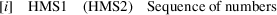The symbols have the following meaning:

• [i]: index of S in G.

• HMS1: short Hermann–Mauguin symbol of S, referred to the coordinate system and setting of G; this symbol may be unconventional.

• (HMS2): conventional short Hermann–Mauguin symbol of S, given only if HMS1 is not in conventional short form.

• Sequence of numbers: coordinate triplets of G retained in S. The numbers refer to the numbering scheme of the coordinate triplets of the general position. For the centred layer groups the following abbreviations are used:

 Block I (all translations retained). Number +: coordinate triplet given by Number, plus that obtained by adding the centring translation (1/2, 1/2, 0) of G. (Numbers) +: the same as above, but applied to all Numbers between parentheses. Block IIa (not all translations retained). Number + (1/2, 1/2, 0): coordinate triplet obtained by adding the translation (1/2, 1/2, 0) to the triplet given by Number. (Numbers) + (1/2, 1/2, 0): the same as above, but applied to all Numbers between parentheses.

#### Examples

 (1) G: Layer group c211 (L10)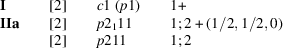where the numbers have the following meaning: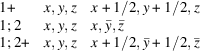(2) G: Rod group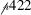(R30)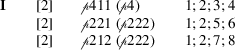The HMS1 symbol in each of the three subgroups S is given in the tetragonal coordinate system of the group G. In the first case,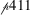is not the conventional short Hermann–Mauguin symbol and a second conventional symbol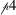is given. In the latter two cases, since the subgroups are orthorhombic rod groups, a second conventional symbol of the subgroup in an orthorhombic coordinate system is given.

#### 1.2.15.1.2. Block IIb

| top | pdf |

Whereas in blocks I and IIa every maximal subgroup S of G is listed, this is no longer the case for the entries of block IIb. The information given in this block is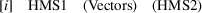The symbols have the following meaning:

 [i]: index of S in G. HMS1: Hermann–Mauguin symbol of S, referred to the coordinate system and setting of G; this symbol may be unconventional. (Vectors): basis vectors of S in terms of the basis vectors of G. No relations are given for basis vectors which are unchanged. (HMS2): conventional short Hermann–Mauguin symbol, given only if HMS1 is not in conventional short form.

#### Examples

 (1) G: Rod group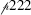(R13)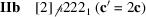There are two subgroups which obey the same basis-vector relation. Apart from the translations of the enlarged cell, the generators of the subgroups, referred to the basis vectors of the enlarged cell, are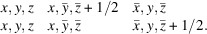(2) G: Layer group pm21b (L28)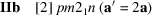This entry represents two subgroups whose generators, apart from the translations of the enlarged cell, are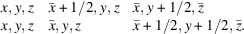The difference between the two subgroups represented by the one entry is due to the different sets of symmetry operations of G which are retained in S. This can also be expressed as different conventional origins of S with respect to G: the two subgroups in the first example above are related by a translation c/4 of the origin, and the two subgroups in the second example by a/4.

#### 1.2.15.2. Maximal isotypic subgroups and enantiomorphic subgroups of lowest index

| top | pdf |

Another set of klassengleiche subgroups is that listed under IIc, i.e. the subgroups S which are of the same or of the enantiomorphic subperiodic group type as G. Again, one entry may correspond to more than one isotypic subgroup:

 (a) As in block IIb, one entry may correspond to two isotypic subgroups whose difference can be expressed as different conventional origins of S with respect to G. (b) One entry may correspond to two isotypic subgroups of equal index but with cell enlargements in different directions which are conjugate subgroups in the affine normalizer of G. The different vector relationships are given, separated by or' and placed within one pair of parentheses; cf. example (2).

#### Examples

 (1) G: Rod group(R13)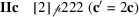This entry corresponds to two isotypic subgroups. Apart from the translations of the enlarged cell, the generators of the subgroups are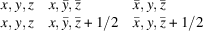(2) G: Layer group pmm2 (L23)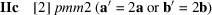This entry corresponds to four isotypic subgroups, two with the enlarged cell with a′ = 2a and two with the enlarged cell with b′ = 2b. The generators of these subgroups are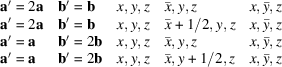(3) G: Rod group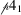(R24)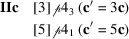Listed here are both the maximal isotypic subgroupand the maximal enantiomorphic subgroup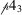, each of lowest index.

#### 1.2.15.3. Minimal non-isotypic non-enantiomorphic supergroups

| top | pdf |

If G is a maximal subgroup of a group H, then H is called a minimal supergroup of G. Minimal supergroups are again subdivided into two types, the translationengleiche or t supergroups I and the klassengleiche or k supergroups II. For the t supergroups I of G, the listing contains the index [i] of G in H and the conventional Hermann–Mauguin symbol of H. For the k supergroups II, the subdivision between IIa and IIb is not made. The information given is similar to that for the subgroups IIb, i.e. the relations between the basis vectors of group and supergroup are given, in addition to the Hermann–Mauguin symbols of H. Note that either the conventional cell of the k supergroup H is smaller than that of the subperiodic group G, or H contains additional centring translations.

#### Example: G: Layer group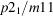(L15)

Minimal non-isotypic non-enantiomorphic supergroups: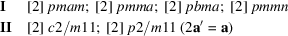Block I lists  pmam,  pmma and  pmmn. Looking up the subgroup data of these three groups one finds  p21/m11. Block I also lists  pbma. Looking up the subgroup data of this group one finds  p121/m1 (p21/m11). This shows that the setting of pbma does not correspond to that of p21/m11 but rather to p121/m1. To obtain the supergroup H referred to the basis of p21/m11, the basis vectors a and b must be interchanged. This changes pbma to pmba, which is the correct symbol of the supergroup of p21/m11.

Block II contains two entries: the first where the conventional cells are the same with the supergroup having additional centring translations, and the second where the conventional cell of the supergroup is smaller than that of the original subperiodic group.

#### 1.2.15.4. Minimal isotypic supergroups and enantiomorphic supergroups of lowest index

| top | pdf |

No data are listed for supergroups IIc, because they can be derived directly from the corresponding data of subgroups IIc.

#### Example: G: Rod group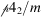(R29)

The maximal isotypic subgroup of lowest index ofis found in block IIc:(c′ = 3c). By interchanging c′ and c, one obtains the minimal isotypic supergroup of lowest index, i.e.(3c′ = c).

### References

International Tables for Crystallography (2005). Vol. A. Space-group symmetry, edited by Th. Hahn. Heidelberg: Springer. [Previous editions: 1983, 1987, 1992, 1995 and 2002. Abbreviated as IT A (2005).]